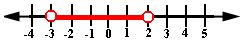## Definition Of Inequality

An inequality is a mathematical sentence that uses symbols such as <, ≤,="">, or ≥ to compare two quantities.

### Example of Inequality

5 < d="">< 7,="" x="" ≥="" -="" 1,="">2 ≤ b, - 12 > - 13 are few examples of inequalities

### Solved Example on Inequality

#### Ques: Pick an appropriate graph for the inequality - 3 < x="" and="" x="">< 2="">##### Choices:

A. Figure 1
B. Figure 2
C. Figure 3
D. None of the aboveStep 4: So, Figure 3 represents the given inequality.